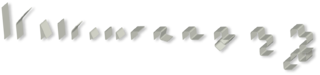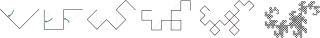Seeking Opportunities
Contact Me

# The Dragon Curve

Not dogma, just what I'm learning and thinking about right now.
Comments and feedback are welcome on Mastodon.

"If you're thinking without writing, then you just think you're thinking."
Leslie Lamport

The Dragon Curve, pictured above, is an example of a “self-similar fractal curve” (Wikipedia). Though that definition sounds oddly redundant to me–self-similarity is a defining characteristic of all fractals. I was introduced to the Dragon Curve while in high school by a magazine article that I can no longer recall. I don’t remember now how this article came to my attention, but I believe the original source was this much older article from Scientific American. I was fascinated at how such a beautiful shape could be created by iterating on a simple set of rules. This was my first introduction to fractals and I have been fascinated by them ever since. Let’s look at how the Dragon Curve fractal is created.

## How the Dragon Curve is made

There are many ways to think about the Dragon Curve, but I believe the original article described it as being discovered by repeatedly folding a piece of paper in half in the same direction, and then unfolding it, with each crease opened to a 90° angle. Looking at the paper edge-on would reveal the start of the Dragon Curve. The illustrations of this process below (and many others) may be found in the Wikipedia article on the Dragon Curve.

Following is what it might look like to create a Dragon Curve by actually folding paper. Unfortunately, as Britney Gallivan would tell you, there is a limit to how many times you can fold a piece of paper. :-/If we skip the paper and simply draw the successive iterations, they would look like this:From these images, we can derive the rules for creating a Dragon Curve. Looking at the first image above, we see that the first fold goes to the right. We will label this “turn” R. After a second fold, we would have three turns: RRL. The first four iterations of folds (about as far as we could go with actual paper) would look like this:

R
RRL
RRLRRLL
RRLRRLLRRRLLRLL


Continuing this process reveals a pattern: each iteration is formed by taking the previous iteration, adding an R, and then appending the “mirror image” of the previous iteration. By “mirror image,” I mean in reverse order and with the “rights” and “lefts” flip-flopped. For example, the “mirror image” of the fourth iteration seen above would be: RRLRRLLLRRLLRLL. Therefore, we could find the fifth iteration by taking the fourth one, adding R, and then adding this mirror image, like so:

RRLRRLLRRRLLRLL + R + RRLRRLLLRRLLRLL = RRLRRLLRRRLLRLLRRRLRRLLLRRLLRLL


What’s so interesting about the Dragon Curve? Well, it has a lot of interesting properties. First, although the curve often folds back to touch itself, it never crosses itself (this could be intuited from its paper-folding origins–the paper can never physically cross itself). Also, those seemingly jagged edges mesh perfectly when tiled, which means they fit together like puzzle pieces that can completely cover a two-dimensional surface! Or, as Wikipedia describes it, the Dragon Curve is “a non-self-crossing space-filling curve.” (0_o) Pretty sure there should be a comma in there somewhere . . . oh, what useful purpose does it have? I have no idea . . . but it sure is pretty! ;-)

## The Dragon Curve Meets the Apple ][e

Okay, so what did I do with this? Why, I drew pretty pictures on the screen of an Apple ][e! (Yes, that’s how Apple spelled it.) First, I generated long strings of Rs and Ls, then I drew increasing iterations of the Dragon to the “Hi-Res” screen (280x192 pixels!!) until . . . it broke. As it turns out, strings in Apple’s Integer BASIC were limited to 255 characters. So, how many iterations of the Dragon Curve could I generate with one string before hitting a *** STR OVFL ERR? As we can see above, the lists of turns seem to grow at a predictable rate: 1, 3, 7, 15. This seems to imply that each iteration $$n$$ will have a turn count of $$2^n-1$$. Or, as I learned, that I could fit precisely eight iterations ($$2^{8} - 1$$) in a 255 character string.

Well, eight iterations didn’t fill my Hi-Res screen! How could I go further? One solution was to make more strings and have the renderer “page” through the strings as needed. The problem with this approach is that the curve’s exponential growth quickly ate up the available memory. I think my favorite approach was to generate the 8th iteration, and then treat that string as a unit to generate further iterations. That is, I started a new string in which I could represent the 8th iteration with the letter N, add an R, and then add an M (for the mirror image of N) to create a new, three-character string representing the 9th iteration: NRM. The rendering routine knew that N represented the 8th iteration “unit,” and the M represented the mirror image of N. In this way, I could keep going for another eight iterations–more than enough to fill the Apple’s screen and, as it turns out, exhaust my teenage attention span.

## The Dragon Curve meets Javascript

Fast forward many (many) years and I found myself learning Javascript and playing around with the canvas element introduced in HTML5. I really wanted to make something cool and pretty. My friend, Heather, could draw amazing things using a canvas element, including portraits. However, I am virtually talent-free when it comes to most things artistic, so I decided to try using math. I turned to my old friend the Dragon Curve.

This time, I didn’t want to mess around with generating strings or arrays to create the curve. I thought, there must be a way to calculate any arbitrary turn in the curve. I was sure that if I just stared at the problem a pattern would emerge . . . Okay, so I stared way longer than anyone would consider to be healthy . . . but I was right, a pattern did emerge! Lets look at the fourth iteration of the curve that we calculated above. Remember that is has 15 turns. I will reproduce it below, with each turn numbered.

                  1 1 1 1 1 1
1 2 3 4 5 6 7 8 9 0 1 2 3 4 5

R R L R R L L R R R L L R L L


I stared at this sequence for a long time, but nothing made sense to me. It was only when I had the idea to stagger the odd and even turns that I started to see the shape of something. Do you see it?

                  1 1 1 1 1 1
1 2 3 4 5 6 7 8 9 0 1 2 3 4 5

R   L   R   L   R   L   R   L
R   R   L   R   R   L   L


The first thing I noticed through my blurry vision was that the odd turns simply alternated between R and L. I could work with that! But what the hell were the even turns doing? It dawned on me suddenly that the even turns were a copy of the Dragon Curve itself! In other words, if I looked only at the fourth iteration above, the even-numbered turns represent the seven turns that make up the previous (third) iteration! (0_0) That means, if we calculated the curve to infinite turns, the even-numbered turns would also contain a complete, infinite copy of the curve. Wild!

What to do with our new knowledge? Well, this article is already long enough, so I’ll save my discussion of the algorithm I derived from this for another article. To make my pretty picture, I decided to explore what a Dragon Curve would look like if I animated it on a canvas element by cycling the angle of the “turns” from 0° to 90° and back to zero. That was cool–it looked like the curve was coiling into place from a straight line, and then uncoiling back into a straight line. Then I drew four curves at once, radiating from a single point. That was cool too–you could see how all four curves locked together perfectly when the angles reached 90 degrees, and then immediately sprang apart again. What else could I do? Well, I decided to make them all spin around the origin as they coiled and uncoiled. And that is how I ended up with this: